# Breadth First Traversal and Depth First Traversal

Amit Kumar Ghosh  10 min read
15 Jun 2023
330 Views

## Introduction

Are you looking to brush up on your data structure knowledge? If so, then understanding the basics of Breadth First Traversal and Depth First Traversal are essential. This article will provide you with a comprehensive overview of these traversal methods, taking the time to explain what they are and why they're important in data structures. By the end of the article, you'll understand how each algorithm works and when it's best utilized perfect for any aspiring software engineer! So let’s start exploring Breadth First Traversal and Depth First Traversal today!

## What is Breadth First Traversal in data structure?

Breadth-first traversal (BFS) is a graph traversal algorithm that explores all the vertices of a graph or tree in breadth-first order. Breadth First Traversal in data structure starts at a given vertex (or root in the case of a tree) and visits all the vertices at the same level before moving to the next level.

Here's a step-by-step explanation of how BFS works:

1. Start by selecting a starting vertex or node.
2. Add the starting vertex to a queue.
3. Mark the starting vertex as visited.
4. While the queue is not empty, do the following steps:
• Remove the front vertex from the queue.
• Visit the removed vertex and process it.
• Enqueue all the adjacent vertices of the removed vertex that have not been visited yet.
• Mark each visited adjacent vertex as visited and add it to the queue.
5. Repeat step 4 until the queue is empty.

Breadth First Traversal in data structure uses a queue data structure to keep track of the vertices to visit. By processing vertices in the order they were added to the queue, BFS ensures that all the vertices at the current level are visited before moving on to the next level. This leads to a breadth-first exploration of the graph or tree, hence the name "breadth-first traversal." BFS is often used for tasks like finding the shortest path between two vertices or detecting cycles in a graph. It guarantees that the shortest path between any two vertices is found when the graph is unweighted.

• One way to visualize the BFS algorithm is by using a graph diagram and step-by-step illustrations. Here's an example:

Let's consider the following undirected graph: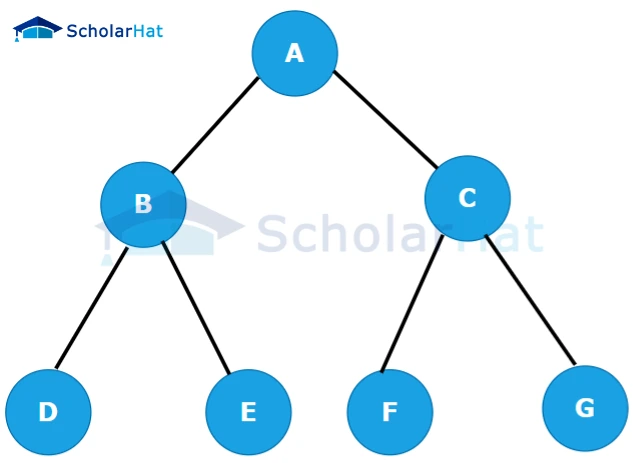We'll start the BFS traversal from vertex A. Here's the step-by-step visualization:

Step 1:

• Start at vertex A and mark it as visited.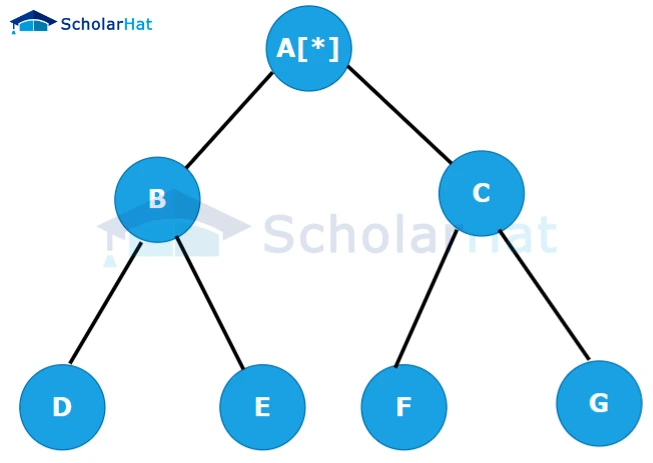Step 2:

• Visit the adjacent vertices of A (B and C) and mark them as visited. Enqueue them in the queue (in any order).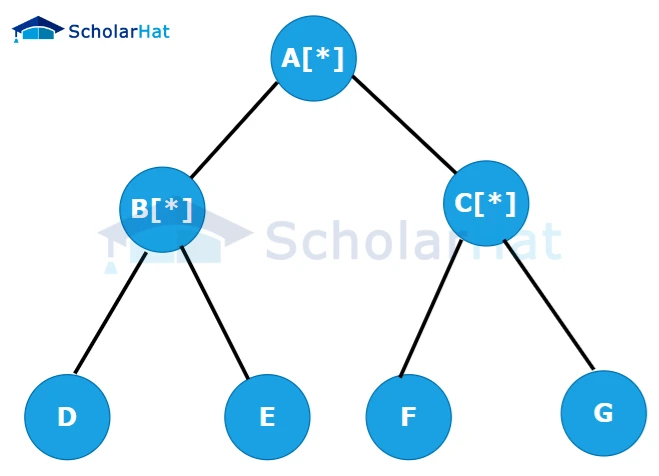Step 3:

• Dequeue vertex B from the queue and visit its adjacent vertices (D and E). Mark them as visited and enqueue them.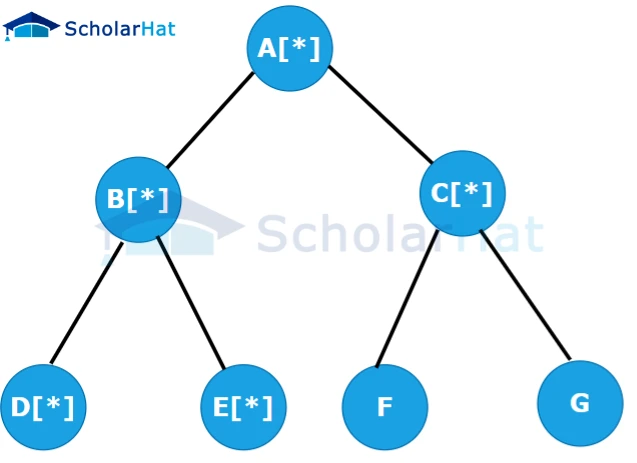Step 4:

• Dequeue vertex C from the queue and visit its adjacent vertices (F and G). Mark them as visited and enqueue them.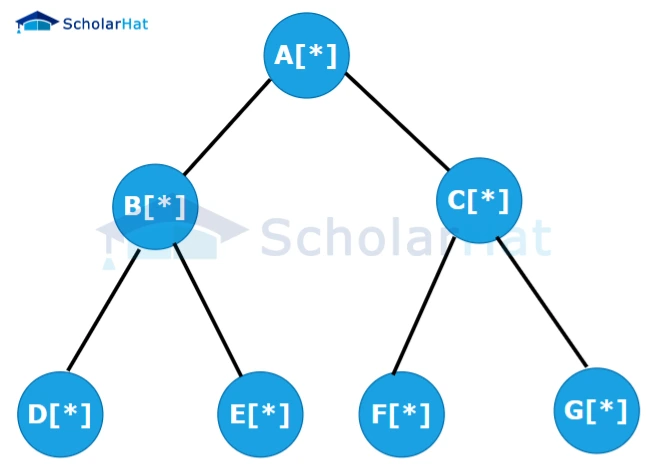Step 5:

• Dequeue vertex D from the queue. As it has no adjacent unvisited vertices, no new vertices are enqueued.Step 6:

• Dequeue vertex E from the queue. Similarly, no new vertices are enqueued.Step 7:

• Dequeue vertex F from the queue. No new vertices are enqueued.Step 8:

• Dequeue vertex G from the queue. No new vertices are enqueued.The BFS traversal of the graph starting from vertex A visits all the vertices in breadth-first order: A, B, C, D, E, F, G.

## What is Depth first traversal in data structure

Depth-first traversal is a graph traversal algorithm that explores as far as possible along each branch before backtracking. Depth first traversal in data structure starts at a given vertex or node in a graph and visits all the adjacent vertices or nodes in a depth-first manner, recursively exploring each neighbor before moving to the next unvisited neighbor.

Here's a step-by-step explanation of the depth-first traversal process:

1. Start with a graph or tree structure and choose a starting vertex or node.
2. Visit the current vertex or node and mark it as visited.
3. Explore the unvisited neighbors of the current vertex or node.
4. Choose one of the unvisited neighbors and repeat steps 2 and 3 from that neighbor.
5. If all neighbors of the current vertex or node have been visited, backtrack to the previous vertex or node and continue exploring any remaining unvisited neighbors.
6. Repeat steps 2-5 until all vertices or nodes have been visited.

The depth-first traversal algorithm follows a depth-first search strategy, where it explores the deepest branches of a graph or tree first before backtracking. This traversal technique is often implemented using recursion, as the recursive calls allow for easy exploration of adjacent vertices or nodes. Depth-first traversal is commonly used in various applications such as graph searching, finding connected components, solving puzzles, and traversing tree structures. It can also be used to generate a depth-first search tree, which represents the traversal path taken during the algorithm execution.

DFS visits all vertices of a connected component and is often implemented using recursion or a stack.

To help visualize the DFS process, let's consider an example

A

/ \

B C

/ \ \

D E F

• We will perform a DFS starting from vertex A. Here's a step-by-step visualization of the DFS process:

Step 1:

• Starting from vertex A, we mark it as visited and explore its adjacent vertices. Let's choose the leftmost unvisited vertex, which is B.

A

/ \

(V)B C

/ \ \

D E F

Step 2:

• We mark vertex B as visited and explore its adjacent vertices. We choose the leftmost unvisited vertex, which is D.

A

/ \

(V)B C

/ \ \

(V)D E F

Step 3:

• Vertex D has no unvisited adjacent vertices, so we backtrack to vertex B and explore its next unvisited adjacent vertex, which is E.

A

/ \

(V)B C

/ \ \

V E F

\

(V)D

Step 4:

• Vertex E has no unvisited adjacent vertices, so we backtrack to vertex B and explore its next unvisited adjacent vertex, which is C.

A

/ \

(V)B (V)C

/ \ \

V E F

\

(V)D

Step 5:

• Vertex C has no unvisited adjacent vertices, so we backtrack to vertex A and explore its next unvisited adjacent vertex, which is F.

A

/ \

(V)B (V)C

/ \ \

V E (V)F

\

(V)D

Step 6:

• Vertex F has no unvisited adjacent vertices, so we backtrack to vertex A. Since all vertices are visited, the DFS process is complete.

The order in which the vertices are visited in this example is A, B, D, E, C, F.

## Difference between BFS and DFS

The basic differences between bfs and dfs are:

 BFS DFS Full form Full form of BFS is Breadth First Search. Full form of DSF Depth First Search. Traversal Order It explores the graph in a breadthward motion, visiting all the nodes at the current level before moving to the next level. It follows the "first in, first out" (FIFO) principle, usually implemented using a queue. It explores the graph in a depthward motion, visiting a node and then recursively exploring its children or neighbors before backtracking. It follows the "last in, first out" (LIFO) principle, usually implemented using a stack or recursion. Memory Usage It requires more memory than DFS because it needs to store all the nodes at the current level in a queue until they are processed. It requires less memory than BFS because it only needs to store a single path from the root to the current node. Completeness It is guaranteed to find a solution (if one exists) in a finite graph with bounded branching factor, as long as the solution is at a shallow depth. It explores all possible nodes at each depth level before moving deeper. It may not find a solution if the search space is infinite or the solution is located at a deep level. It can get stuck in an infinite path or go down a deep branch before exploring other branches. Time Complexity The time complexity of BFS is O(V + E), where V is the number of vertices (nodes) and E is the number of edges in the graph. It visits every vertex and edge once. The time complexity of DFS is also O(V + E), but DFS tends to go deeper before exploring other branches, so it may be less efficient in practice for certain graphs. Applications It is commonly used to find the shortest path between two nodes in an unweighted graph, to traverse levels of a tree or graph iteratively, or to check for connectivity in a graph. It is often used to solve problems like finding connected components, topological sorting, finding cycles or paths in a graph, or solving puzzles like the maze problem.
##### Summary

Understanding these two types of tree traversal methods is essential to programming; without this knowledge, developers will be unable to approach algorithms correctly and efficiently. Both methods have their uses, with Breadth First Traversal being most useful when a node's degree is known in advance. On the other hand, Depth First Traversal is best suited for finding all adjacent nodes very quickly. Whether you need to search through trees or experiment with algorithms, it's crucial to know which method you should use for the task at hand. So, make sure to really dive into Breadth First Traversal and Depth First Traversal in data structure to become a better programmer and craft better applications!

Similar Articles